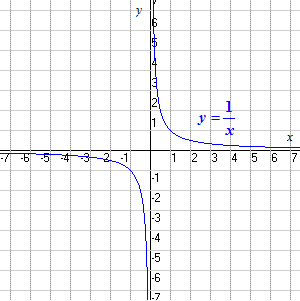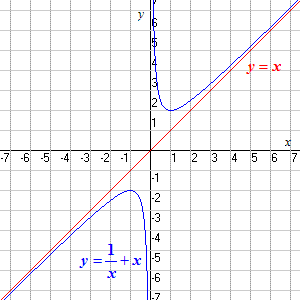# Asymptotes

An asymptote to a curve is a straight line which the curve approaches without crossing it. If we go sufficiently far along the line, the curve becomes arbitrarily close.

A simple example is the graph of $y=\frac{1}{x}$ . This curve has both the $x$ -axis and the $y$ -axis as asymptotes.As $x$ goes to infinity, the graph approaches the $x$ -axis from above; as $x$ goes to negative infinity, the graph approaches the $x$ -axis from below.

Similarly, as $y$ goes to infinity, the graph approaches the $y$ -axis from the right; as $y$ goes to negative infinity, the graph approaches the $y$ -axis from the left.

Diagonal asymptotes are also possible; for instance, the graph of has the line $y=x$ as an asymptote. (The $y$ -axis is also an asymptote.)